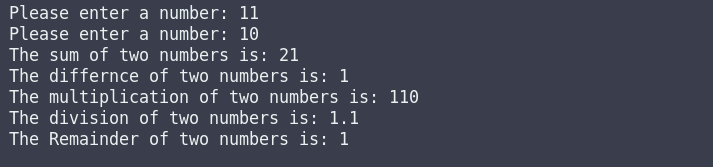Write a c++ program in which you have to take input from the user two numbers using global variables and create six functions one for set data, second for addition, third for difference, fourth for division, fifth for multiplication, and sixth for the remainder and print the addition, subtraction, division, multiplication, and the remainder of those numbers.

Sample Program

The sum of two numbers is: 21

The difference between the two numbers is: 1

The multiplication of two numbers is: 110

The division of two numbers is: 1.1

The Remainder of two numbers is: 1

#include <iostream>
using namespace std;

// Declaring Varibles
float num1;
float num2;

// Function for to setdata
void setData(){
cin>>num1;
cin>>num2;
}

cout<<"The sum of two numbers is: "<<num1+num2<<endl;
}

void Substraction(){
cout<<"The differnce between two numbers is: "<<num1-num2<<endl;
}

void Multiplication(){
cout<<"The multiplication of two numbers is: "<<num1*num2<<endl;
}

void Division(){
cout<<"The division of two numbers is: "<<num1/num2<<endl;
}

void Remainder(){
cout<<"The Remainder of two numbers is: "<<int(num1)%int(num2)<<endl;
}

int main()
{
setData();cpp programming exercise with solution